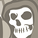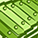# QlikView App Dev

Discussion Board for collaboration related to QlikView App Development.

Announcements
Welcome to Qlik Community! Check out our new navigation! FIND OUT MORE
cancel
Showing results for
Did you mean:Contributor III

## Help with skipping an i in a for loop (or moving to next i) with If statement

Hi all,

I am trying to integrate to Google Maps. Whilst I've managed to do that, I need to handle records where there is no address. Some records do not have an address as I've mashed all the FACTS into one.

What I am doing is peeking inside a field, and, if it's null, I want to move to the next i in the for loop.

If there's an address, then move to the table.

I've mucked around with a few options but none seem to work.

Be gentle, I'm just a beginner.

Here's what I have: what I end up with is a GMAP call that fails as address is often null.

```let noRows = NoOfRows('Facts')-1;

for i=1 to \$(noRows)
//let a=peek('Pt_FNAME',\$(i),'Facts');
//let b=peek('Pt_LNAME',\$(i),'Facts');
//let c=peek(‘ProductSold’,\$(i),'Facts');

GeocodeResponse:
status,
//'\$(a)' as Pt_FNAME,
//'\$(b)' as Pt_LNAME,
//'\$(c)' as ProductSold,

([result/geometry/location/lat]) as latitude,
([result/geometry/location/lng]) as longitude
next i;

```

Here's what I have come up with in the for loop, but I get a script error. Basically, the logic is: if address is null, try the next i, if it's not null, carry on to the GeocodeResponse.

```let noRows = NoOfRows('Facts')-1;

for i=1 to \$(noRows)

//     let a=peek('Pt_FNAME',\$(i),'Facts');
//       let b=peek('Pt_LNAME',\$(i),'Facts');
//let c=peek(‘ProductSold’,\$(i),'Facts');
if IsNull (\$address)) Then
Exit FOR
//also have tried Next i;
Else
```
1 Solution

Accepted SolutionsChampion

Hi Tim,

Try this,

Let noRows = NoOfRows('Facts')-1;

for i=1 to \$(noRows)

//let a=peek('Pt_FNAME',\$(i),'Facts');

//let b=peek('Pt_LNAME',\$(i),'Facts');

//let c=peek(‘ProductSold’,\$(i),'Facts');

If Not isnull(address) Then

GeocodeResponse:

status,

//'\$(a)' as Pt_FNAME,

//'\$(b)' as Pt_LNAME,

//'\$(c)' as ProductSold,

([result/geometry/location/lat]) as latitude,

([result/geometry/location/lng]) as longitude

ELSE

ENDIF

next i;

3 RepliesChampion

Hi Tim,

Try this,

Let noRows = NoOfRows('Facts')-1;

for i=1 to \$(noRows)

//let a=peek('Pt_FNAME',\$(i),'Facts');

//let b=peek('Pt_LNAME',\$(i),'Facts');

//let c=peek(‘ProductSold’,\$(i),'Facts');

If Not isnull(address) Then

GeocodeResponse:

status,

//'\$(a)' as Pt_FNAME,

//'\$(b)' as Pt_LNAME,

//'\$(c)' as ProductSold,

([result/geometry/location/lat]) as latitude,

([result/geometry/location/lng]) as longitude

ELSE

ENDIF

next i;Contributor III
Author

Winner, winner, chicken dinner. (Except if you're plant powered!)

Thank you so very much Tamil!

And now, onto the troublesome graph plotting!Champion

Ha ha ha. Good luck and have a happy day Tim!.